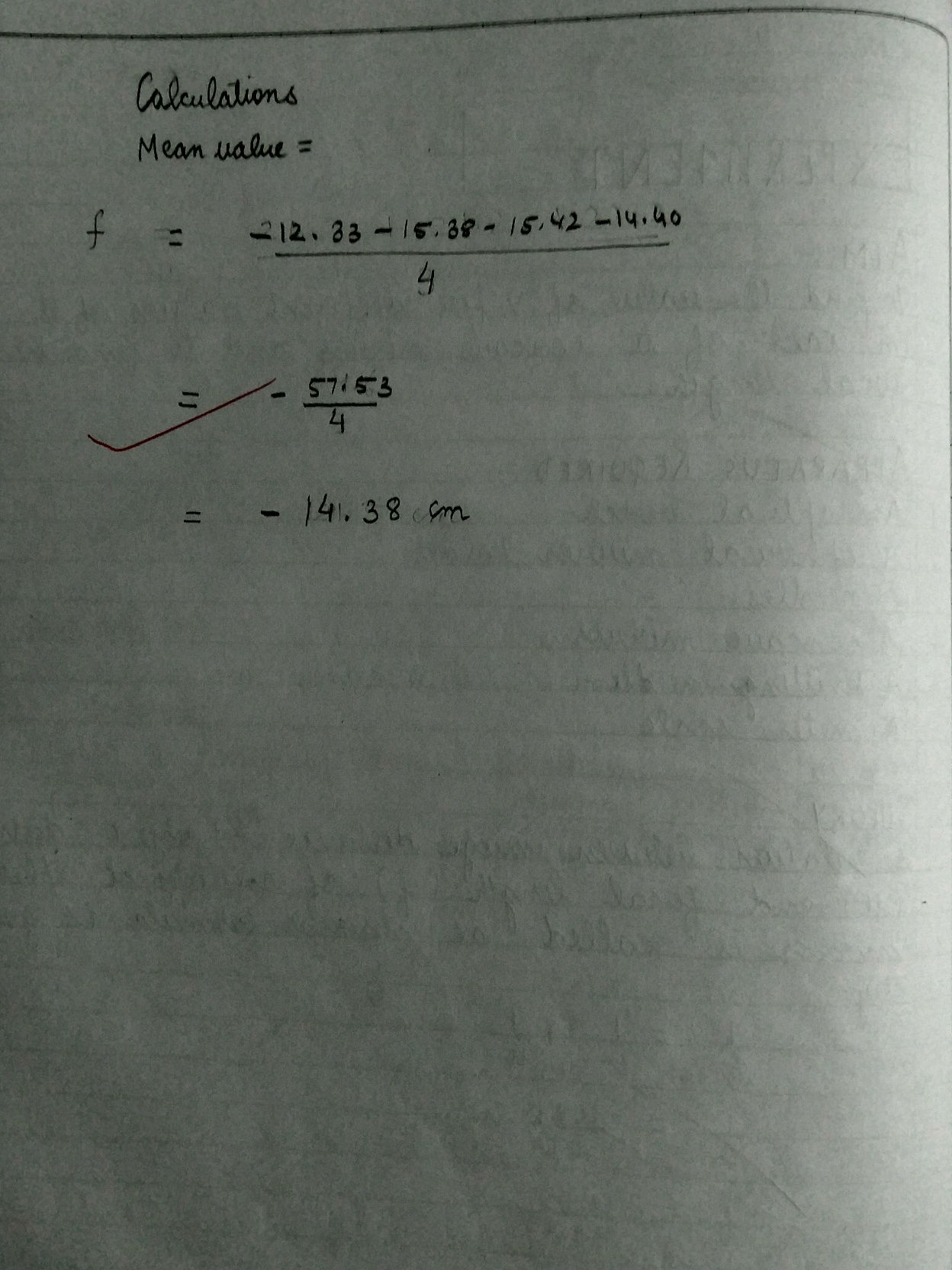<

# To find the value of V for different values of u in case of a concave mirror and to find the focal length.## Class 12Physics Practicals List

` Practical : 1`
; ` Practical : 2`
; ` Practical : 3`
; ` Practical : 4`
; ` Practical : 5`
; ` Practical : 6`
; ` Practical : 7`
; ` Practical : 8`
; ` Practical : 9`
; ` Practical : 10`
; ` Practical : 11`
; ` Practical : 12`
; ` Practical : 13`
; ` Practical : 14`
; ` Practical : 15`
; ` Practical : 16`
; ` Practical : 17`
; ` Practical : 18`
; ` Practical : 19`
; ` Practical : 20`
; ` Practical : 21`
; ` Practical : 22`
;